# AP Calculus AB Practice Test 53

### Test Information10 questions20 minutes

Calculator Disallowed

1. The graph of function fis shown below. Which of the following is true for fon the interval (a, b)?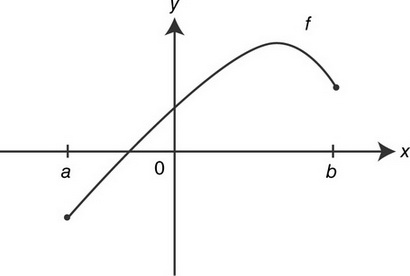I. The function f is differentiable on (a, b).

II. There exists a number kon (a, b) such that f′(k) = 0.

III. f″ > 0 on (a, b).

2. The velocity function of a moving particle on the x-axis is given as v(t) = t2&- 3t&- 10. For what positive values of tis the particle’s speed increasing?

3. The graph of fconsists of two line segments and a semicircle for &- 2 ≤ x≤ 2 as shown below. What is the value of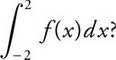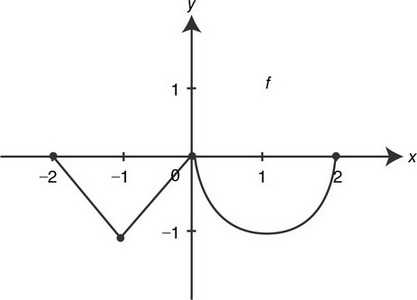4. What is the average value of the function y= 3 cos(2x) on the interval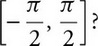5. If f(x) = |x3|, what is the value of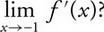6. A spherical balloon is being inflated. At the instant when the rate of increase of the volume of the sphere is four times the rate of increase of the radius, the radius of the sphere is

7. If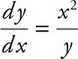and at x= 0, y= 4, a solution to the differential equation is

8. The area of the region enclosed by the graph of x= y2&- 1 and they-axis is

9. The graph off′, the derivative of f, is shown below. At which value of xdoes the graph fhave a horizontal tangent?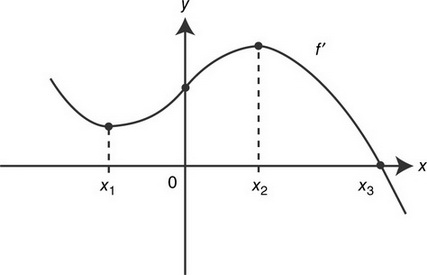10. If f(x) = &- |x&- 3|, which of the following statements about fis true?

I. fis differentiable at x= 3.

II. fhas an absolute minimum at x= 3.

III. fhas a point of inflection at x= 3.